# Liquid Solution - JEE Advanced Previous Year Questions with Solutions

JEE Advanced Previous Year Questions of Chemistry with Solutions are available at eSaral. Practicing JEE Advanced Previous Year Papers Questions of Chemistry will help the JEE aspirants in realizing the question pattern as well as help in analyzing weak & strong areas. Simulator Previous Years JEE Advance Questions
Q. The Henry’s law constant for the solubility of $\mathrm{N}_{2}$ gas in water at 298 K is 1.0 × $10^{5}$ atm. The mole fraction of N2 in air is 0.8. The number of moles of $\mathrm{N}_{2}$ from air dissolved in 10 moles of waterat 298 K and 5 atm pressure is- (A) $4.0 \times 10^{-4}$ (B) $4.0 \times 10^{-5}$ (C) $5.0 \times 10^{-4}$ (D) $4.0 \times 10^{-5}$ [JEE 2009]
Ans. (A) $\mathrm{P}_{\mathrm{N}_{2}}=\mathrm{K}_{\mathrm{H}} \mathrm{X}_{\mathrm{N}_{2}}$ $\mathrm{Y}_{\mathrm{N}_{2}} \cdot \mathrm{P}_{\mathrm{T}}=\mathrm{K}_{\mathrm{H}} \times \mathrm{N}_{2}$ $0.8 \times 5=1 \times 10^{5} \times \frac{\mathrm{n}}{\mathrm{n}+10}$ $4=10^{5} \times \frac{\mathrm{n}}{10}$ $\mathrm{n}=4 \times 10^{-4}$
Q. For a dilute solution containing 2.5 g of a non-volatile non-electrolyte solute in 100 g of water, the elevation in boiling point at 1 atm pressure is $2^{\circ} \mathrm{C}$. Assuming concentration of solute is much lower than the concentration of solvent, the vapour pressure (mm of Hg) of the solution is-(take $\left.\mathrm{K}_{\mathrm{b}}=0.76 \mathrm{K} \mathrm{kg} \mathrm{mol}^{-1}\right)$ (A) 724             (B) 740            (C) 736             (D) 718 [JEE 2011]
Ans. (A)
Q. The freezing point (in °C) of a solution containing 0.1 g of $\mathrm{K}_{3}\left[\mathrm{Fe}(\mathrm{CN})_{6}\right]$ (Mol. Wt. 329) in 100 g of water $\left(\mathrm{K}_{\mathrm{f}}=1.86 \mathrm{K} \mathrm{kg} \mathrm{mol}^{-1}\right)$ is – (A) $-2.3 \times 10^{-2}$ (B) $-5.7 \times 10^{-2}$ (C) $-5.7 \times 10^{-3}$ (D)-1.2 \times 10^{-2} [JEE 2011]
Ans. (A) $\mathrm{T}_{\mathrm{f}}^{\prime}=\mathrm{T}_{\mathrm{f}}-\Delta \mathrm{T}_{\mathrm{f}}=\mathrm{T}_{\mathrm{f}}-\mathrm{i} \mathrm{K}_{\mathrm{f}} \cdot \mathrm{m}$ $=0^{\circ} \mathrm{C}-4 \times 1.86 \times \frac{0.1 / 329}{100 / 1000}$ $=-0.023^{\circ} \mathrm{C}=-2.3 \times 10^{2} \mathrm{C}$
Q. For a dilute solution containing 2.5 g of a non-volatile non-electrolyte solute in 100 g of water, the elevation in boiling point at 1 atm pressure is 2^{\circ} \mathrm{C}. Assuming concentration of solute is much lower than the concentration of solvent, the vapour pressure (mm of Hg) of the solution is \text { (take }\left.\mathrm{K}_{\mathrm{b}}=0.76 \mathrm{K} \mathrm{kg} \mathrm{mol}^{-1}\right) (A) 724 (B) 740 (C) 736 (D) 718 [JEE 2012]
Ans. (A) $\Delta \mathrm{T}_{\mathrm{b}}=\mathrm{K}_{\mathrm{b}} \cdot \mathrm{m}$ $2=0.76 \times \frac{\mathrm{n}}{100 / 1000}$ $\mathrm{n}=0.263 \mathrm{mol}$ $\mathrm{P}_{\mathrm{S}}=\mathrm{P}^{\circ} \mathrm{x}_{\text {solvent }}=760 \times \frac{\frac{100}{18}}{\frac{100}{18}+0.263}=760 \times \frac{5.55}{5.82}=724.7$ torr
Q. Benzene and naphthalene form an ideal solution at room temperature. For this process, the true statement(s) is(are) (A) $\Delta \mathrm{G}$ is positive (B) $\Delta S_{\text {system }}$ is positive (C) $\Delta \mathrm{S}_{\text {surroundings }}=0$ (D) $\Delta \mathrm{H}=0$ [J-Adv. 2013]
Ans. (B,C,D)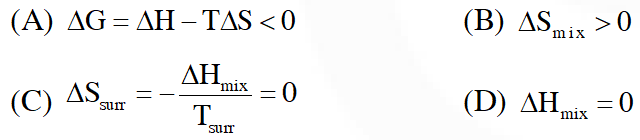Q. A compound $\mathrm{H}_{2} \mathrm{X}$ with molar weight of 80 g is dissolved in a solvent having density of $0.4 \mathrm{g} \mathrm{mL}^{-1}$, Assuming no change in volume upon dissolution, the molality of a 3.2 molar solution is [JEE-Adv. 2014]
Ans. 8 $\mathrm{m}=\frac{3.2 \mathrm{mol}}{0.4 \mathrm{Kg}}=8 \mathrm{mol} / \mathrm{kg}$
Q. If the freezing point of a 0.01 molal aqueous solution of a cobalt (III) chloride-ammonia complex (which behaves as a strong eletrolyte) is $-0.0558^{\circ} \mathrm{C}$ , the number of chloride (s) in the coordination sphere of the complex is- $\left[\mathrm{K}_{\mathrm{f}} \text { of water }=1.86 \mathrm{K} \mathrm{kg} \mathrm{mol}^{-1}\right]$ [JEE-Adv. 2015]
Ans. 1 0.0558 = i × 1.86 × 0.01 i = 3 $\left[\mathrm{Co}\left(\mathrm{NH}_{3}\right)_{5} \mathrm{Cl}\right] \mathrm{Cl}_{2}$
Q. Mixture(s) showing positive deviation from Raoult's law at $35^{\circ}$C is (are) (A) carbon tetrachloride + methanol (B) carbon disulphide + acetone (C) benzene + toluene (D) phenol + aniline [JEE - Adv. 2016]
Ans. (A,C) (A) H-bonding of methanol breaks when $\mathrm{CCl}_{4}$ is added so bonds become weaker, resulting positive deviation. (B) Mixing of polar and non-polar liquids will produce a solution of weaker interaction, resulting positive deviation (C) Ideal solution (D) –ve deviation because stronger H-bond is formed.
Q. For a solution formed by mixing liquids L and M, the vapour pressure of L plotted against the mole fraction of M in solution is shown in the following figure, Here $\mathrm{x}_{\mathrm{L}}$ and $\mathbf{X}_{\mathrm{M}}$ represent mole fractions of L and M, respectively, in the solution. the correct statement(s) applicable to this system is(are) -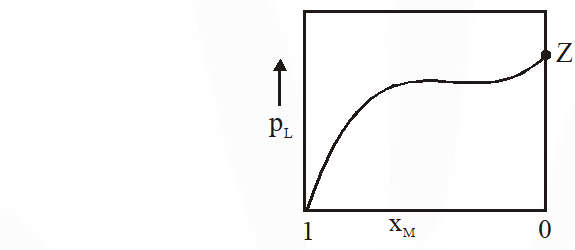(A) Attractive intramolecular interactions between L–L in pure liquid L and M–M in pure liquid M are stronger than those between L–M when mixed in solution (B) The point $Z$ represents vapour pressure of pure liquid $\mathrm{M}$ and Raoult's law is obeyed when $\quad \mathrm{x}_{\mathrm{L}} \rightarrow 0$ (C) The point Z represents vapour pressure of pure liquid L and Raoult's law is obeyed when $\mathrm{x}_{\mathrm{L}} \rightarrow 1$ (D) The point Z represents vapour pressure of pure liquid M and Raoult's law is obeyed from $\mathrm{x}_{\mathrm{L}}=0$ to $\mathrm{x}_{\mathrm{L}}=1$ [JEE - Adv. 2017]
Ans. (A,C)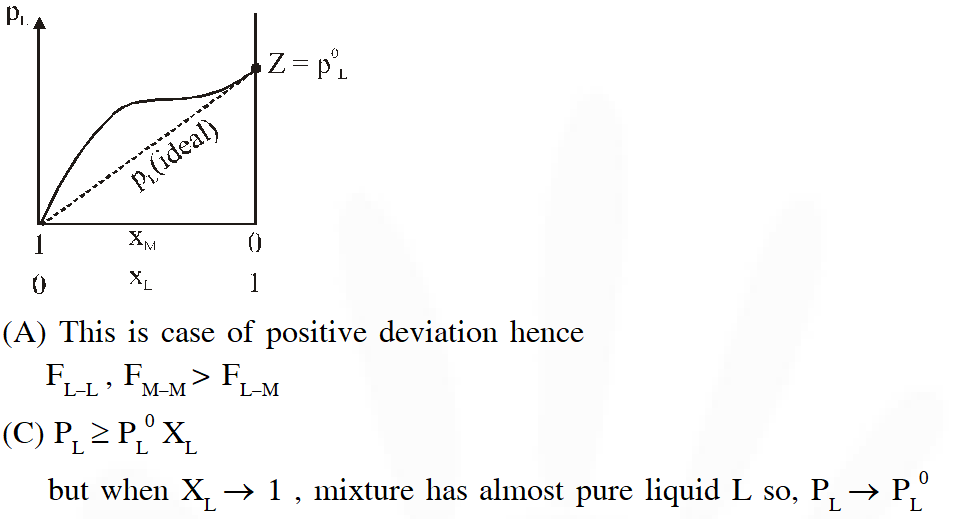Q. Pure water freezes at 273 K and 1 bar. The addition of 34.5 g of ethanol to 500 g of water changes the freezing point of the solution. Use the freezing point depression constant of water as 2 K kg $\mathrm{mol}^{-1}$ . The figures shown below represents plots of vapour pressure (V.P.) versus temperature (T). [Molecular weight of ethanol is 46 g $\mathrm{mol}^{-1}$] Among the following, the option representing change in the freezing point is –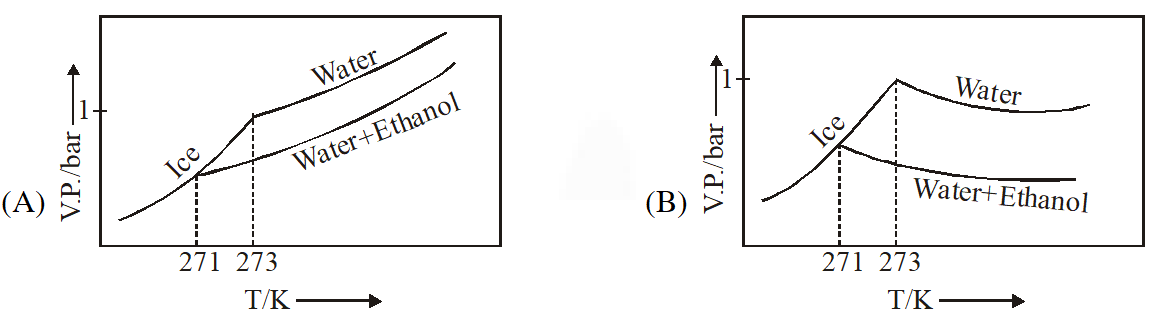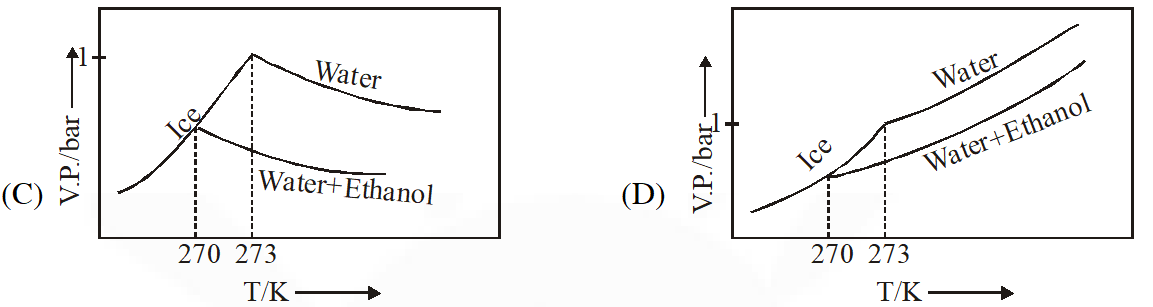[JEE - Adv. 2017]
Ans. (D) Ethanol should be considered non volatile as per given option $\Delta \mathrm{T}_{\mathrm{f}}=\mathrm{K}_{\mathrm{f}} \times \mathrm{m}$ $\Delta \mathrm{T}_{\mathrm{f}}=2 \times \frac{34.5}{46 \times 0.5}=3 \mathrm{K}$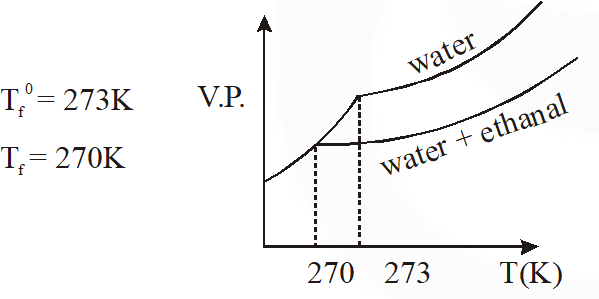Q. Liquids A and B form ideal solution over the entire range of composition. At temperature T, equimolar binary solution of liquids A and B has vapour pressure 45 Torr. At the same temperature, a new solution of A and B having mole fractions $\mathbf{X}_{\mathrm{A}}$ and $\mathrm{x}_{\mathrm{B}}$, respectively, has vapour pressure of 22.5 Torr. The value of $\mathrm{x}_{\mathrm{A}} / \mathrm{x}_{\mathrm{B}}$ in the new solution is____. (given that the vapour pressure of pure liquid A is 20 Torr at temperature T) [JEE - Adv. 2018]
Ans. 19 $45=\mathrm{P}_{\mathrm{A}}^{\mathrm{o}} \times \frac{1}{2}+\mathrm{P}_{\mathrm{B}}^{\mathrm{o}} \times \frac{1}{2}$$\mathrm{P}_{\mathrm{A}}^{\mathrm{o}}+\mathrm{P}_{\mathrm{B}}^{\circ}=90 \ldots \ldots(1)$ given $\mathrm{P}_{\mathrm{A}}^{\circ}=20$ torr $\mathrm{P}_{\mathrm{B}}^{\circ}=70 \mathrm{torr}$ $\Rightarrow 22.5$ torr $=20 \mathrm{x}_{\mathrm{A}}+70\left(1-\mathrm{x}_{\mathrm{A}}\right)$ $=70-50 \mathrm{x}_{\mathrm{A}}$ $\mathrm{x}_{\mathrm{A}}=\left(\frac{70-22.5}{50}\right)=0.95$ $\mathrm{x}_{\mathrm{B}}=0.05$ So $\frac{\mathrm{x}_{\mathrm{A}}}{\mathrm{x}_{\mathrm{B}}}=\frac{0.95}{0.05}=19$
Q. The plot given below shows P–T curves (where P is the pressure and T is the temperature) for two solvents X and Y and isomolal solutions of NaCl in these solvents. NaCl completely dissociates in both the solvents.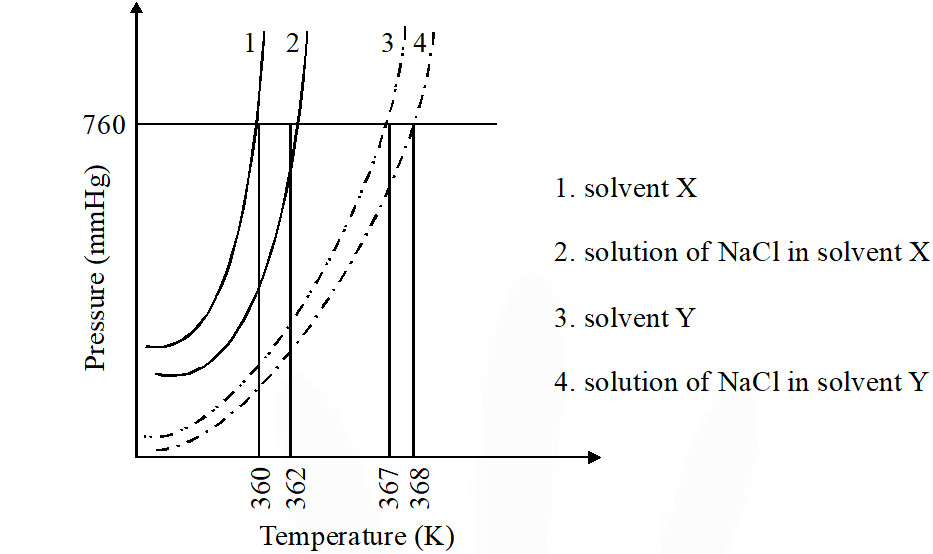On addition of equal number of moles a non-volatile solute S in equal amount (in kg) of these solvents, the elevation of boiling point of solvent X is three times that of solvent Y. Solute S is known to undergo dimerization in these solvents. If the degree of dimerization is 0.7 in solvent Y, the degree of dimerization in solvent X is ___. [JEE - Adv. 2018]
Ans. 0.05 From graph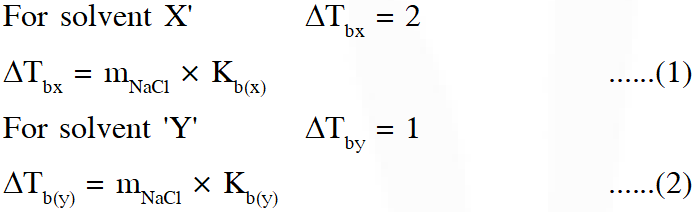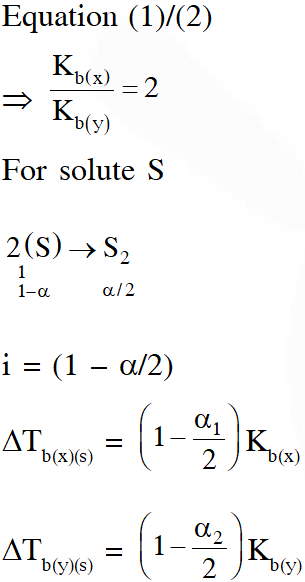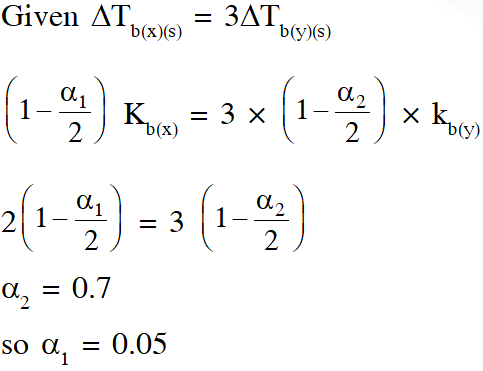Esaral Moderator
May 13, 2022, 10:42 p.m.
sab chutiyapa hai esaral ka.... sab chapa hua hai...dhang se explain toh kr deta
rajesh kshetrapal
June 6, 2021, 2:56 p.m.
jee adv 2016 ans error hai
rajesh kshetrapal
June 6, 2021, 2:30 p.m.
sir, kindly i request to u that pls upload full pyq s of liquid solution
Bamniya
Sept. 25, 2020, 1:11 p.m.
Amazing
Bharath
Sept. 23, 2020, 11:28 a.m.
Excellent
himanshuuu
May 28, 2020, 7:04 p.m.
katai zehr